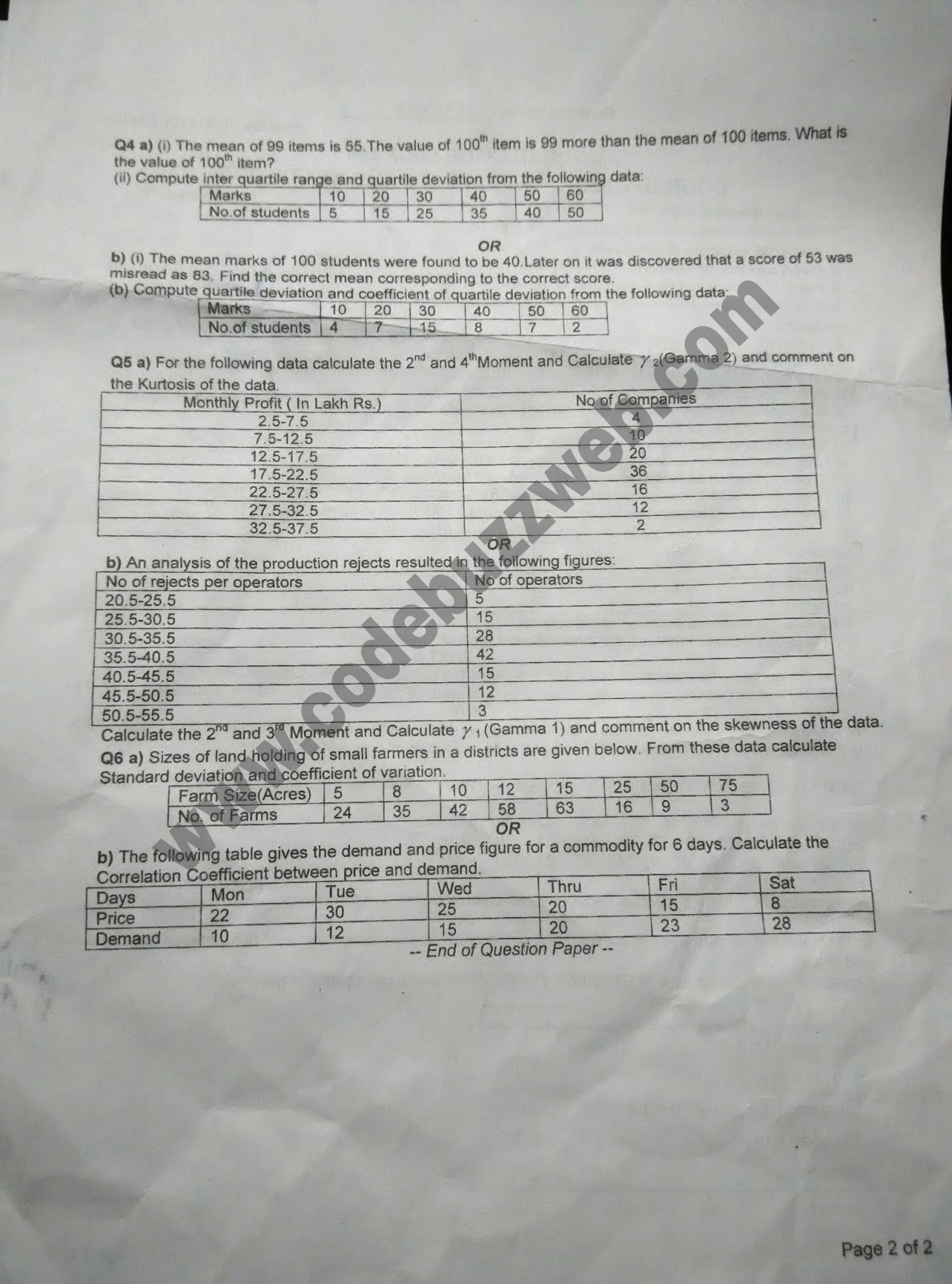# ECE256 : Probability and Statistics End Term Question paper LPU-LPU Question papers | ECE 256 |

Probability and Statistics or also called Statistics and Probability are two related but separate academic disciplines. Statistical analysis often uses probability distributions, and the two topics are often studied together. Wikipedia

ECE256 : Probability and Statistics End Term Question paper-1ECE256 : Probability and Statistics End Term Question paper-2